Next: Soft Iron Sphere in Up: Magnetostatics in Magnetic Media Previous: Permanent Ferromagnets

# Uniformly Magnetized Sphere

Consider a sphere of radius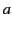, with a uniform permanent magnetization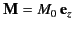, surrounded by a vacuum region. The simplest way of solving this problem is in terms of the scalar magnetic potential introduced in Equation (701). It follows from Equations (703) and (704) that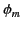satisfies Laplace's equation,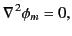(717)

because there is zero volume magnetic charge density in a vacuum, or a uniformly magnetized magnetic medium. However, according to Equation (711), there is a magnetic surface charge density,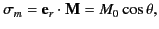(718)

on the surface of the sphere. Here,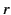and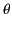are spherical coordinates. One of the matching conditions at the surface of the sphere is that the tangential component of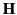must be continuous. It follows from Equation (701) that the scalar magnetic potential must be continuous at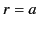, so that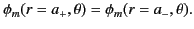(719)

Integrating Equation (703) over a Gaussian pill-box straddling the surface of the sphere yields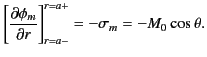(720)

In other words, the magnetic charge sheet on the surface of the sphere gives rise to a discontinuity in the radial gradient of the magnetic scalar potential at.

The most general axisymmetric solution to Equation (718) that satisfies physical boundary conditions atand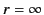is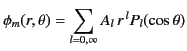(721)

for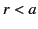, and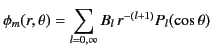(722)

for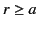. The boundary condition (720) yields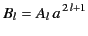(723)

for all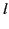. The boundary condition (721) gives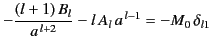(724)

for all, because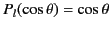. It follows that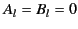(725)

for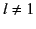, and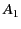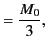(726)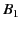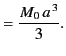(727)

Thus,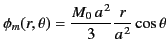(728)

for, and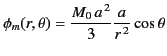(729)

for. Because there is a uniqueness theorem associated with Poisson's equation (see Section 2.3), we can be sure that this axisymmetric potential is the only solution to the problem that satisfies physical boundary conditions at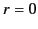and infinity.

In the vacuum region outside the sphere,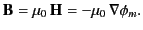(730)

It is easily demonstrated from Equation (730) that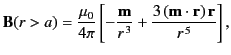(731)

where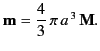(732)

This, of course, is the magnetic field of a magnetic dipole of moment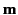. [See Section 5.5.] Not surprisingly, the net dipole moment of the sphere is equal to the integral of the magnetization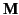(which is the dipole moment per unit volume) over the volume of the sphere.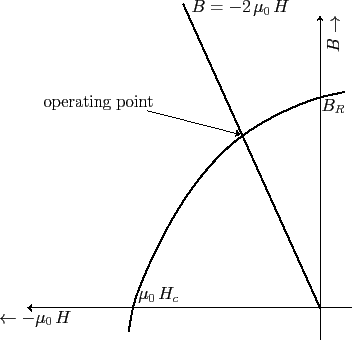Inside the sphere, we have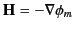and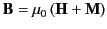, giving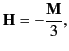(733)

and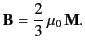(734)

Thus, both theand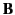fields are uniform inside the sphere. Note that the magnetic intensity is oppositely directed to the magnetization. In other words, thefield acts to demagnetize the sphere. How successful it is at achieving this depends on the shape of the hysteresis curve in the negative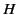and positive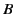quadrant. This curve is sometimes called the demagnetization curve of the magnetic material that makes up the sphere. Figure 4 shows a schematic demagnetization curve. The curve is characterized by two quantities: the retentivity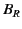(i.e., the residual magnetic field strength at zero magnetic intensity) and the coercivity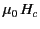(i.e., the negative magnetic intensity required to demagnetize the material. The latter quantity is conventionally multiplied by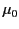to give it the units of magnetic field-strength). The operating point (i.e., the values ofand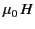inside the sphere) is obtained from the intersection of the demagnetization curve and the curve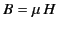. It is clear from Equations (734) and (735) that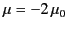(735)

for a uniformly magnetized sphere in the absence of external fields. The magnetization inside the sphere is easily calculated once the operating point has been determined. In fact,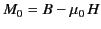. It is clear from Figure 4 that for a magnetic material to be a good permanent magnet it must possess both a large retentivity and a large coercivity. A material with a large retentivity but a small coercivity is unable to retain a significant magnetization in the absence of a strong external magnetizing field.Next: Soft Iron Sphere in Up: Magnetostatics in Magnetic Media Previous: Permanent Ferromagnets
Richard Fitzpatrick 2014-06-27